# 978-0073382395 Chapter 4 Questions and Problems 1-10

Document Type
Homework Help
Book Title
Fundamentals of Corporate Finance Standard Edition 9th Edition
Authors
Stephen Ross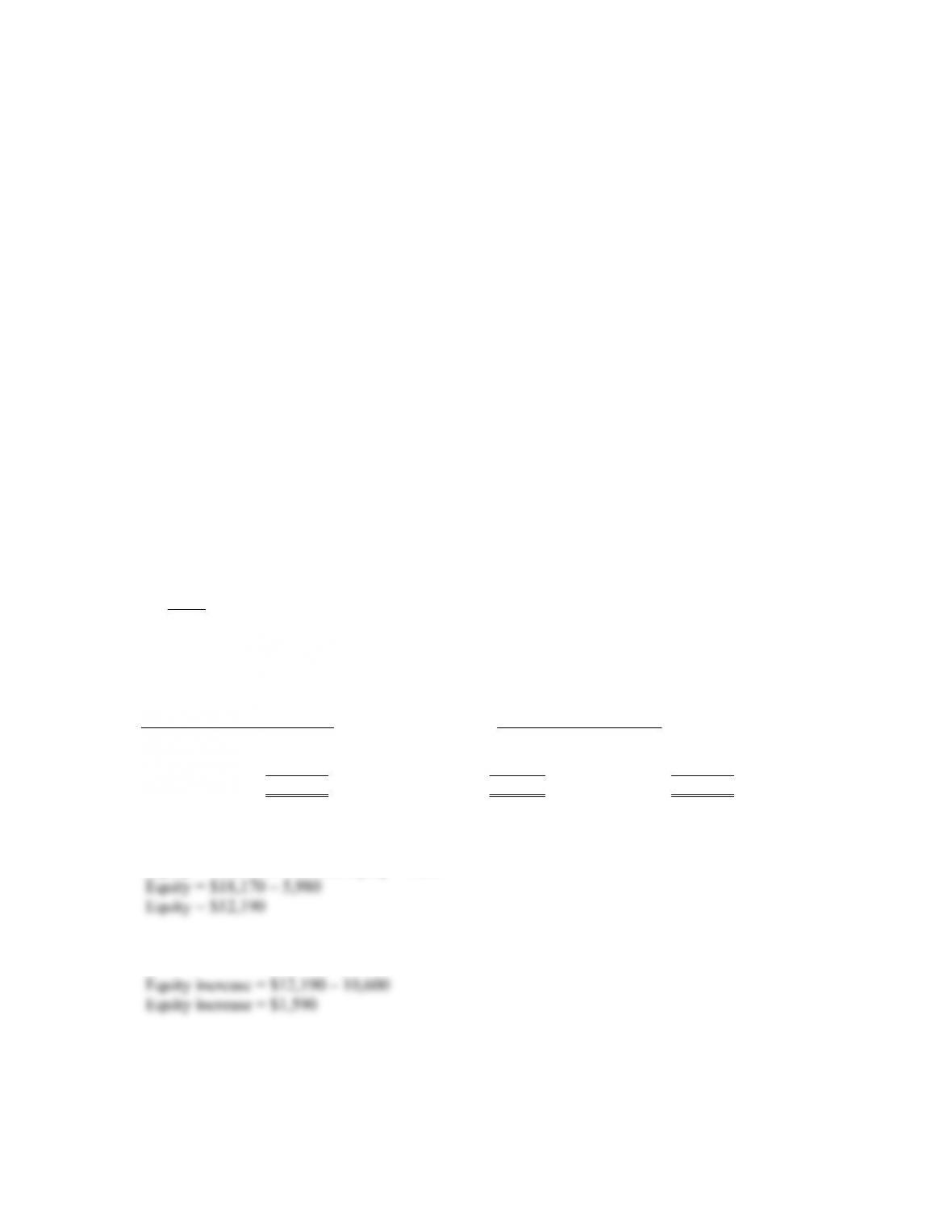B-30 SOLUTIONS
7. Apparently not! In hindsight, the firm may have underestimated costs and also underestimated the
extra demand from the lower price.
8. Financing possibly could have been arranged if the company had taken quick enough action.
Sometimes it becomes apparent that help is needed only when it is too late, again emphasizing the
need for planning.
9. All three were important, but the lack of cash or, more generally, financial resources ultimately
spelled doom. An inadequate cash resource is usually cited as the most common cause of small
10. Demanding cash up front, increasing prices, subcontracting production, and improving financial
resources via new owners or new sources of credit are some of the options. When orders exceed
capacity, price increases may be especially beneficial.
SolutionstoQuestionsandProblems
NOTE: All end of chapter problems were solved using a spreadsheet. Many problems require multiple
steps. Due to space and readability constraints, when these intermediate steps are included in this
solutions manual, rounding may appear to have occurred. However, the final answer for each problem is
found without rounding during any step in the problem.
Basic
1. It is important to remember that equity will not increase by the same percentage as the other assets.
If every other item on the income statement and balance sheet increases by 15 percent, the pro forma
income statement and balance sheet will look like this:
Pro forma income statement Pro forma balance sheet
Sales \$ 26,450 Assets \$ 18,170 Debt \$ 5,980
Costs 19,205 Equity 12,190
Net income \$ 7,245 Total \$ 18,170 Total \$ 18,170
In order for the balance sheet to balance, equity must be:
Equity = Total liabilities and equity – Debt
Equity increased by: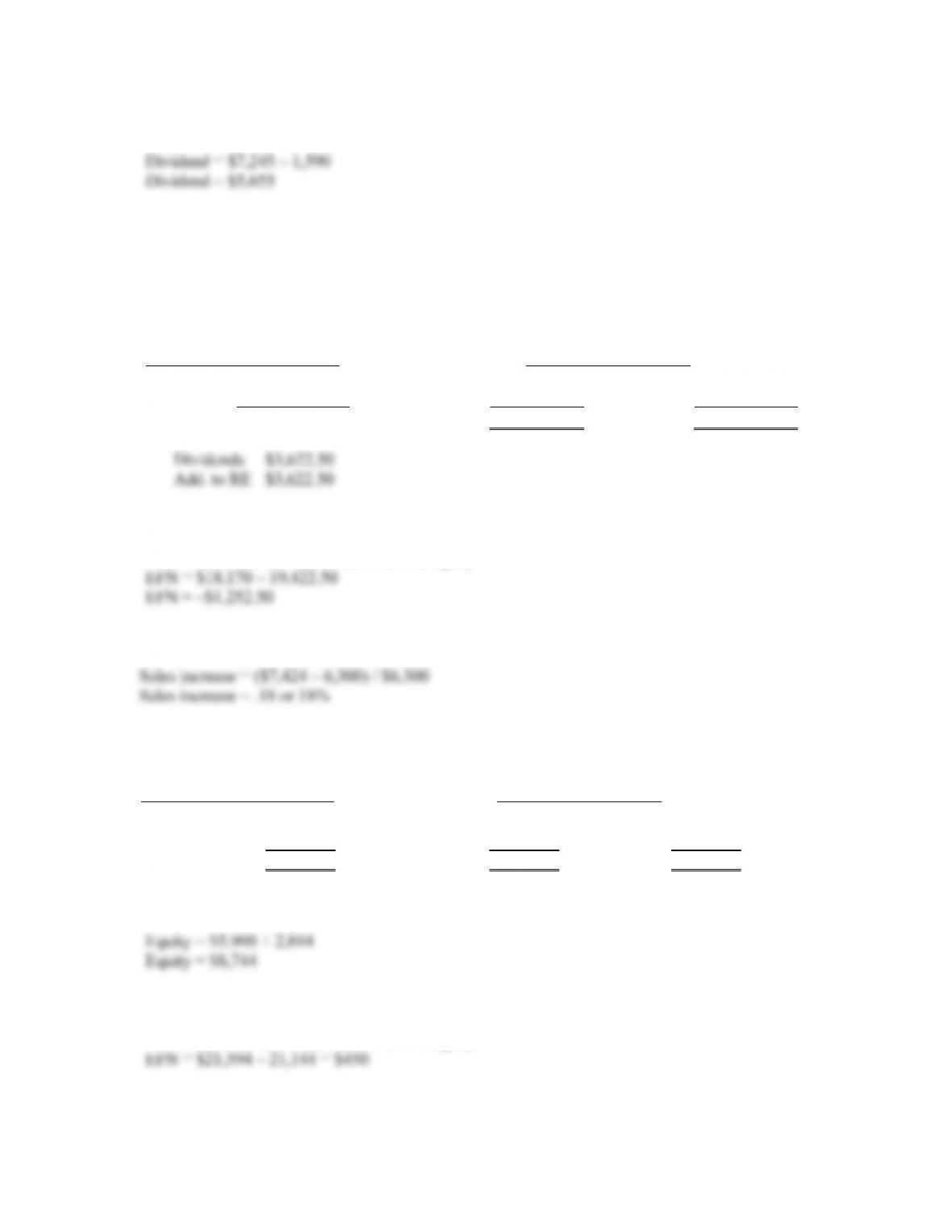CHAPTER 4 B-31
Net income is \$7,245 but equity only increased by \$1,590; therefore, a dividend of:
must have been paid. Dividends paid is the plug variable.
2. Here we are given the dividend amount, so dividends paid is not a plug variable. If the company pays
out one-half of its net income as dividends, the pro forma income statement and balance sheet will
look like this:
Pro forma income statement Pro forma balance sheet
Sales \$26,450.00 Assets \$18,170.00 Debt \$ 5,980.00
Costs 19,205.00 Equity 14,222.50
Net income \$ 7,245.00 Total \$18,170.00 Total \$19,422.50
Note that the balance sheet does not balance. This is due to EFN. The EFN for this company is:
EFN = Total assets – Total liabilities and equity
3. An increase of sales to \$7,424 is an increase of:
Assuming costs and assets increase proportionally, the pro forma financial statements will look like
this:
Pro forma income statement Pro forma balance sheet
Sales \$ 7,434 Assets \$ 21,594 Debt \$ 12,400
Costs 4,590 Equity 8,744
Net income \$ 2,844 Total \$ 21,594 Total \$ 21,144
If no dividends are paid, the equity account will increase by the net income, so:
So the EFN is:
EFN = Total assets – Total liabilities and equity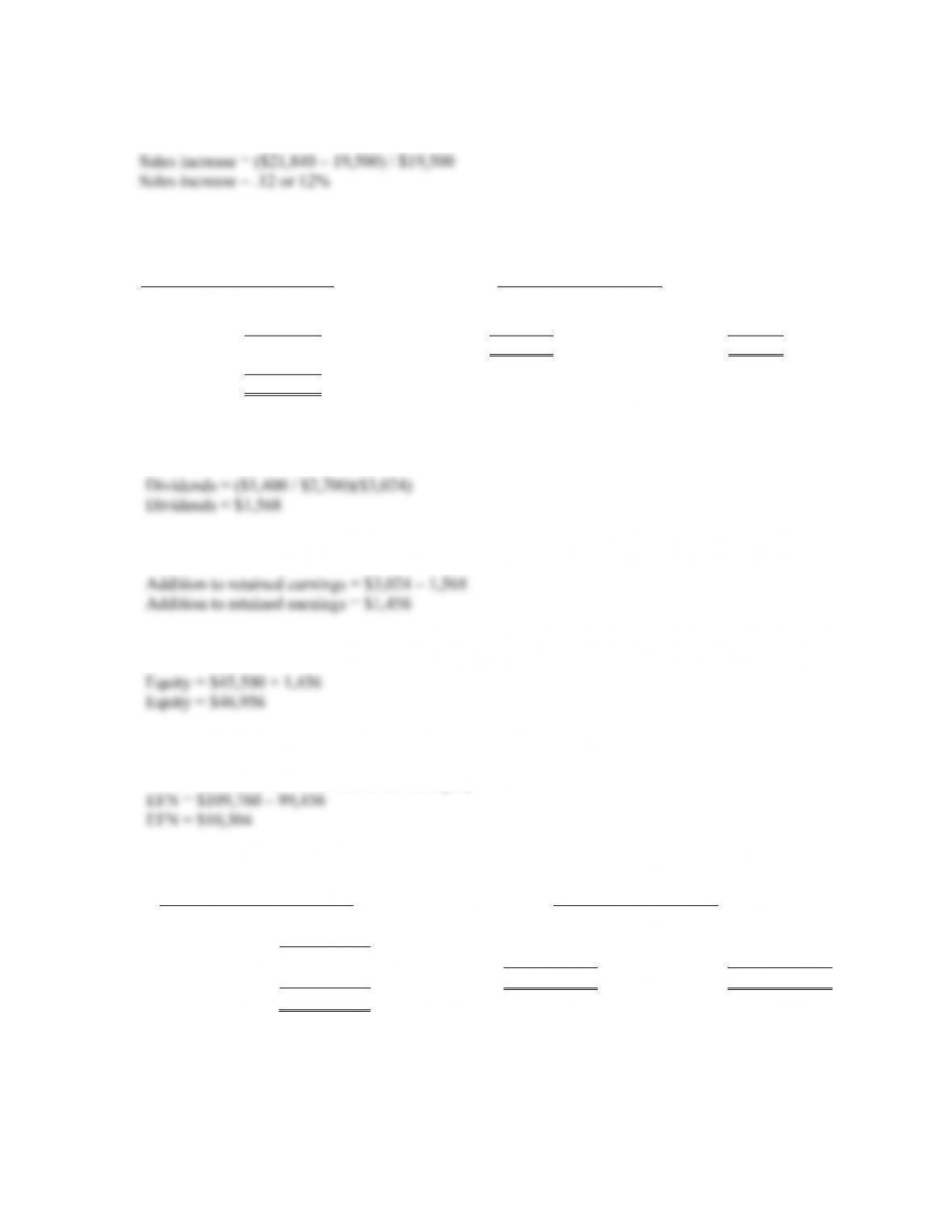B-32 SOLUTIONS
4. An increase of sales to \$21,840 is an increase of:
Assuming costs and assets increase proportionally, the pro forma financial statements will look like
this:
Pro forma income statement Pro forma balance sheet
Sales \$ 21,840 Assets \$109,760 Debt \$52,500
Costs 16,800 Equity 79,208
EBIT 5,040 Total \$109,760 Total \$99,456
Taxes (40%) 2,016
Net income \$ 3,024
The payout ratio is constant, so the dividends paid this year is the payout ratio from last year times
net income, or:
The addition to retained earnings is:
And the new equity balance is:
So the EFN is:
EFN = Total assets – Total liabilities and equity
5. Assuming costs and assets increase proportionally, the pro forma financial statements will look like
this:
Pro forma income statement Pro forma balance sheet
Sales \$4,830.00 CA \$4,140.00 CL \$2,145.00
Costs 3,795.00 FA 9,085.00 LTD 3,650.00
Taxable income \$1,035.00 Equity 6,159.86
Taxes (34%) 351.90 TA \$13,225.00 Total D&E \$12,224.86
Net income \$ 683.10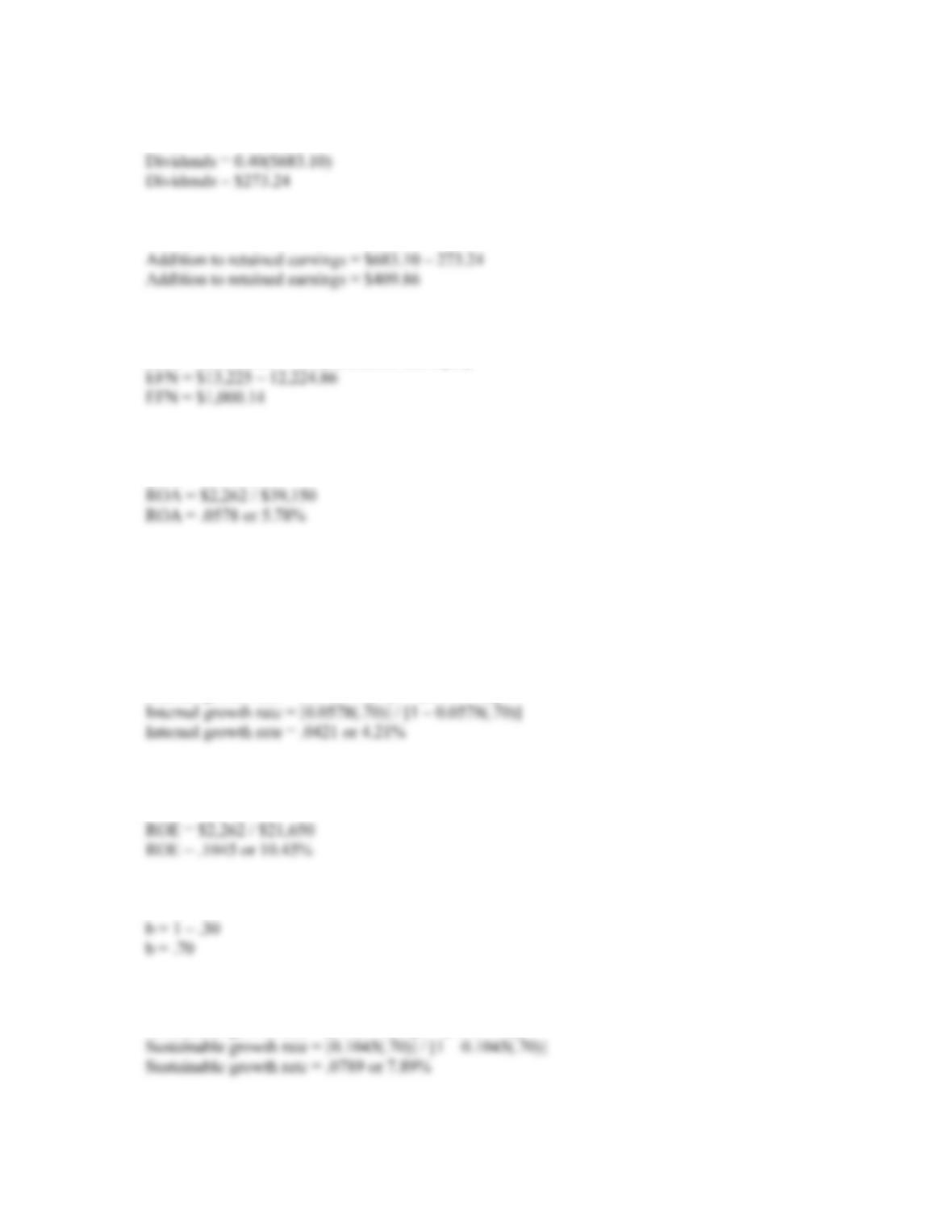CHAPTER 4 B-33
The payout ratio is 40 percent, so dividends will be:
The addition to retained earnings is:
So the EFN is:
EFN = Total assets – Total liabilities and equity
6. To calculate the internal growth rate, we first need to calculate the ROA, which is:
ROA = NI / TA
The plowback ratio, b, is one minus the payout ratio, so:
b = 1 – .30
b = .70
Now we can use the internal growth rate equation to get:
Internal growth rate = (ROA × b) / [1 – (ROA × b)]
7. To calculate the sustainable growth rate, we first need to calculate the ROE, which is:
ROE = NI / TE
The plowback ratio, b, is one minus the payout ratio, so:
Now we can use the sustainable growth rate equation to get:
Sustainable growth rate = (ROE × b) / [1 – (ROE × b)]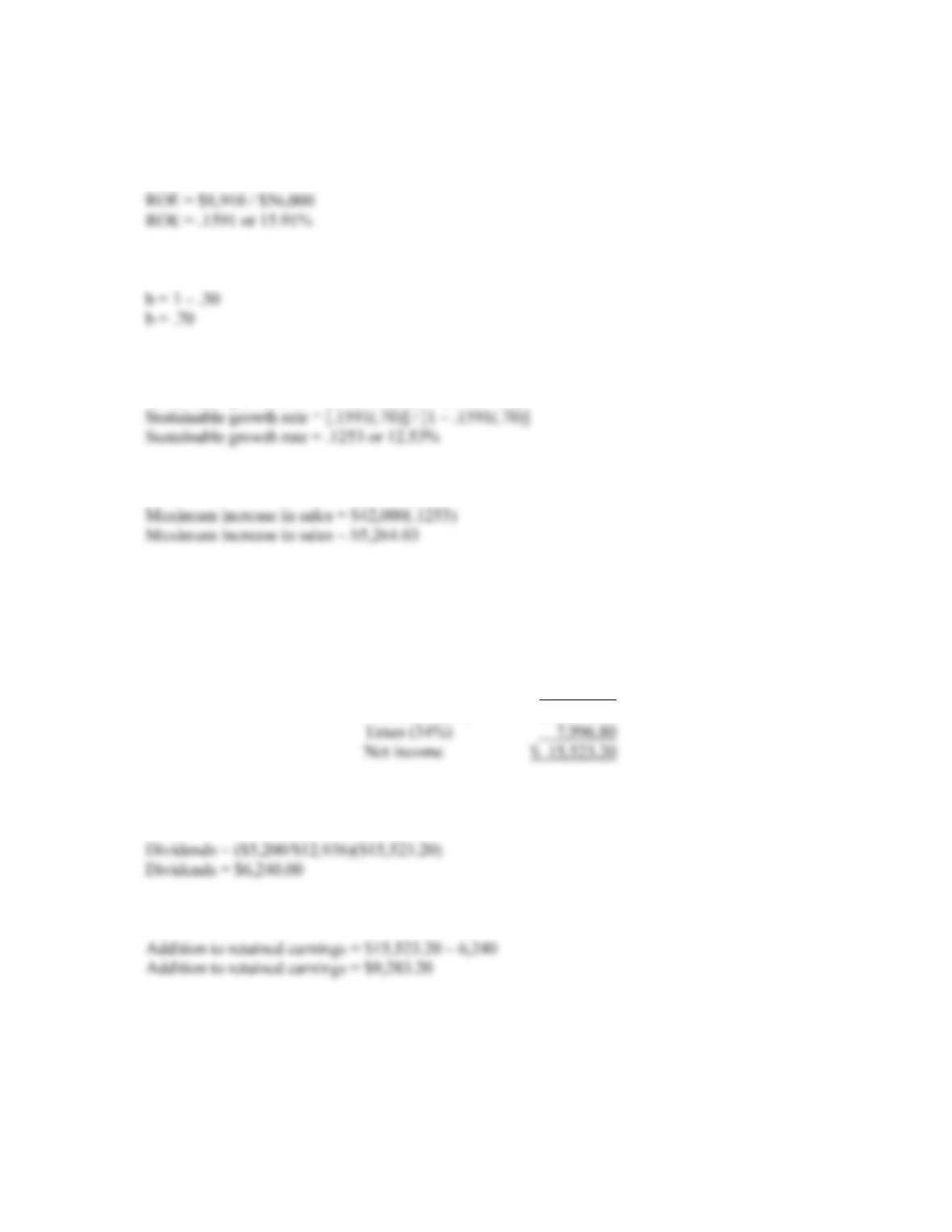B-34 SOLUTIONS
8. The maximum percentage sales increase is the sustainable growth rate. To calculate the sustainable
growth rate, we first need to calculate the ROE, which is:
ROE = NI / TE
The plowback ratio, b, is one minus the payout ratio, so:
Now we can use the sustainable growth rate equation to get:
Sustainable growth rate = (ROE × b) / [1 – (ROE × b)]
So, the maximum dollar increase in sales is:
9. Assuming costs vary with sales and a 20 percent increase in sales, the pro forma income statement
will look like this:
HEIR JORDAN CORPORATION
Pro Forma Income Statement
Sales \$45,600.00
Costs 22,080.00
Taxable income \$23,520.00
The payout ratio is constant, so the dividends paid this year is the payout ratio from last year times
net income, or:
And the addition to retained earnings will be: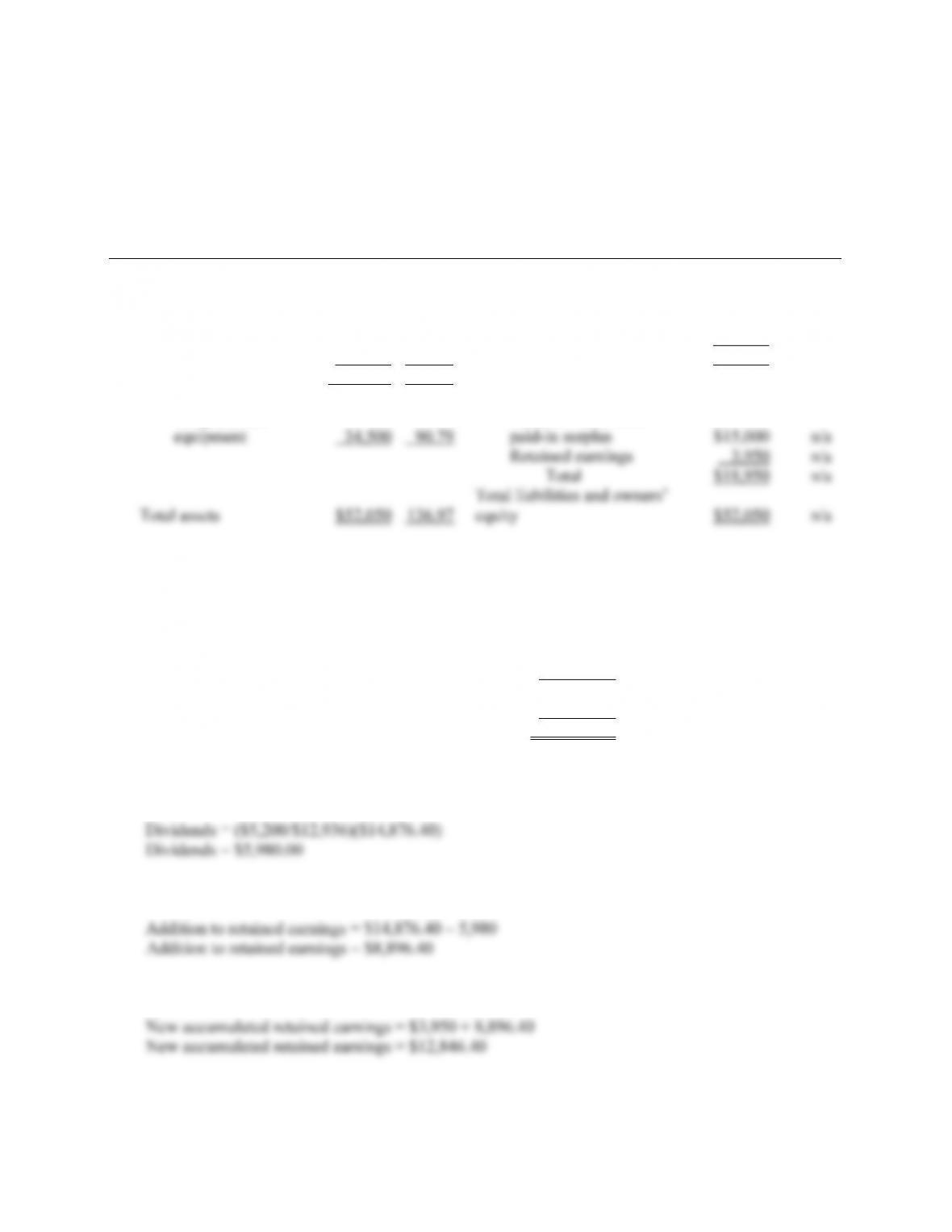CHAPTER 4 B-35
10. Below is the balance sheet with the percentage of sales for each account on the balance sheet. Notes
payable, total current liabilities, long-term debt, and all equity accounts do not vary directly with
sales.
HEIR JORDAN CORPORATION
Balance Sheet
(\$) (%) (\$) (%)
Assets Liabilities and Owners’ Equity
Current assets Current liabilities
Cash \$ 3,050 8.03 Accounts payable \$ 1,300 3.42
Accounts receivable 6,900 18.16 Notes payable 6,800 n/a
Inventory 7,600 20.00 Total \$ 8,100 n/a
Total \$ 17,550 46.18 Long-term debt 25,000 n/a
Fixed assets Owners’ equity
Net plant and Common stock and
11. Assuming costs vary with sales and a 15 percent increase in sales, the pro forma income statement
will look like this:
HEIR JORDAN CORPORATION
Pro Forma Income Statement
Sales \$43,700.00
Costs 21,160.00
Taxable income \$22,540.00
Taxes (34%) 7,663.60
N
et income \$ 14,876.40
The payout ratio is constant, so the dividends paid this year is the payout ratio from last year times
net income, or:
And the addition to retained earnings will be:
The new accumulated retained earnings on the pro forma balance sheet will be: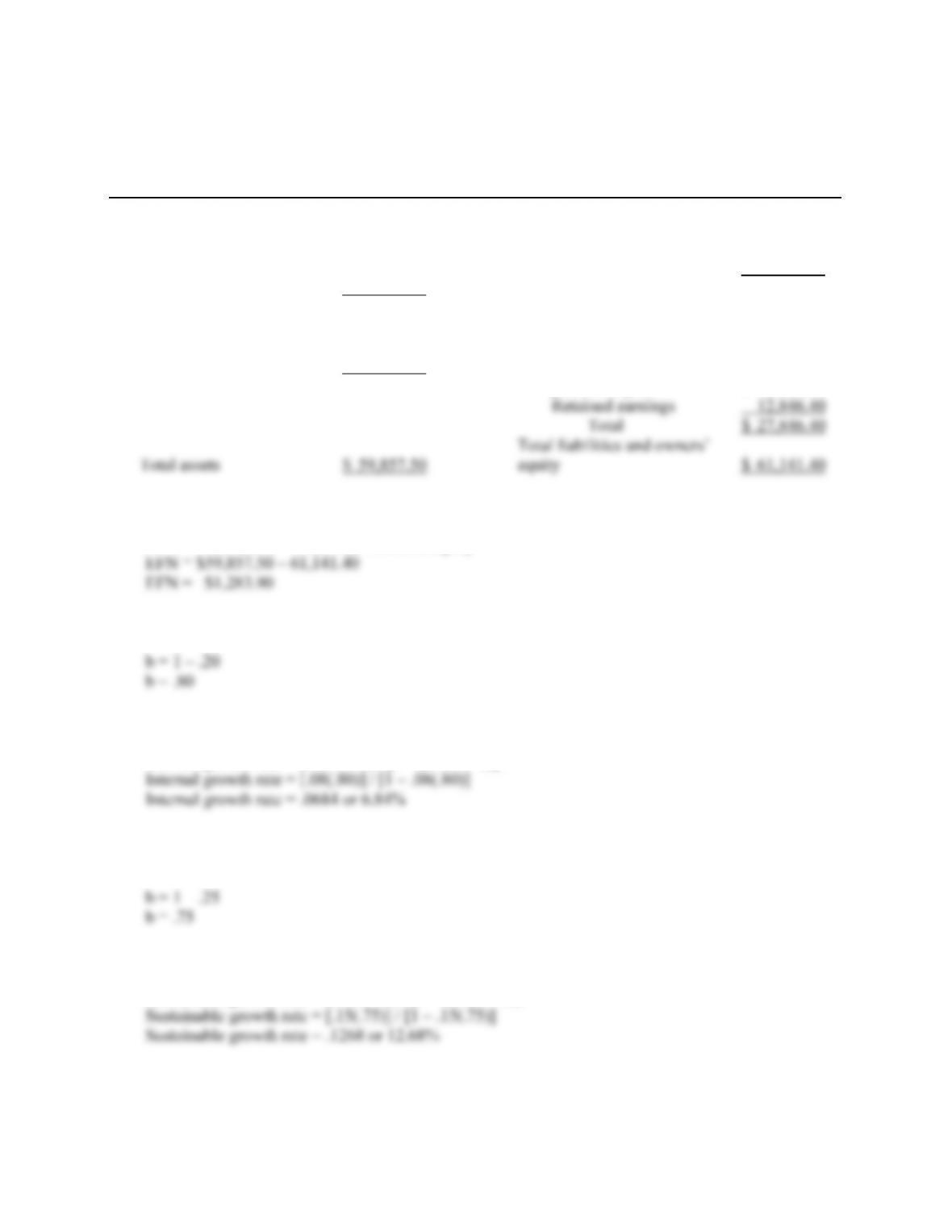B-36 SOLUTIONS
The pro forma balance sheet will look like this:
HEIR JORDAN CORPORATION
Pro Forma Balance Sheet
Assets Liabilities and Owners’ Equity
Current assets Current liabilities
Cash \$ 3,507.50 Accounts payable \$ 1,495.00
Accounts receivable 7,935.00 Notes payable 6,800.00
Inventory 8,740.00 Total \$ 8,295.00
Total \$20,182.50 Long-term debt 25,000.00
Fixed assets
Net plant and Owners’ equity
equipment 39.675.00 Common stock and
paid-in surplus \$ 15,000.00
So the EFN is:
EFN = Total assets – Total liabilities and equity
12. We need to calculate the retention ratio to calculate the internal growth rate. The retention ratio is:
Now we can use the internal growth rate equation to get:
Internal growth rate = (ROA × b) / [1 – (ROA × b)]
13. We need to calculate the retention ratio to calculate the sustainable growth rate. The retention ratio
is:
Now we can use the sustainable growth rate equation to get:
Sustainable growth rate = (ROE × b) / [1 – (ROE × b)]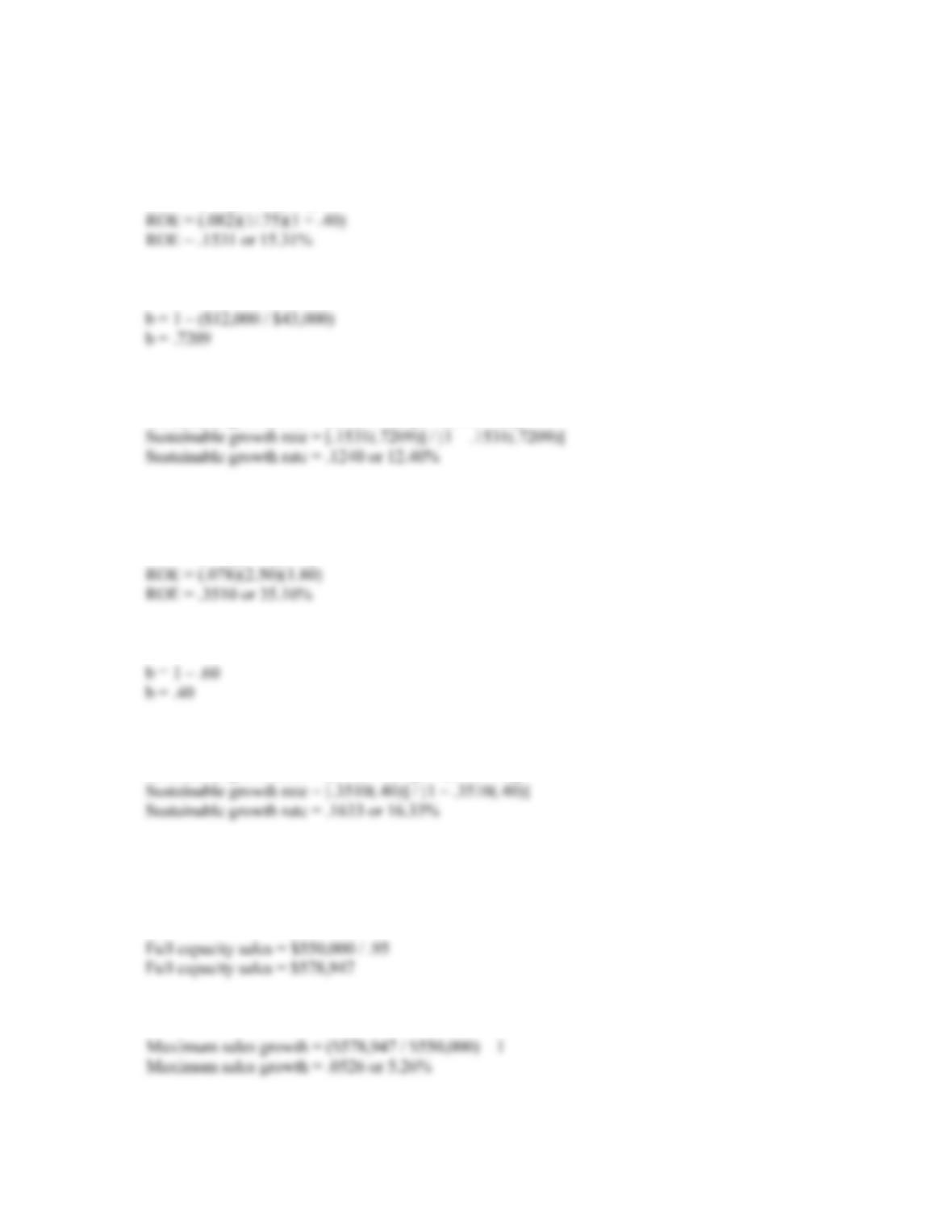CHAPTER 4 B-37
14. We first must calculate the ROE to calculate the sustainable growth rate. To do this we must realize
two other relationships. The total asset turnover is the inverse of the capital intensity ratio, and the
equity multiplier is 1 + D/E. Using these relationships, we get:
ROE = (PM)(TAT)(EM)
The plowback ratio is one minus the dividend payout ratio, so:
Now we can use the sustainable growth rate equation to get:
Sustainable growth rate = (ROE × b) / [1 – (ROE × b)]
15. We must first calculate the ROE using the DuPont ratio to calculate the sustainable growth rate. The
ROE is:
ROE = (PM)(TAT)(EM)
The plowback ratio is one minus the dividend payout ratio, so:
Now we can use the sustainable growth rate equation to get:
Sustainable growth rate = (ROE × b) / [1 – (ROE × b)]
Intermediate
16. To determine full capacity sales, we divide the current sales by the capacity the company is currently
using, so:
The maximum sales growth is the full capacity sales divided by the current sales, so: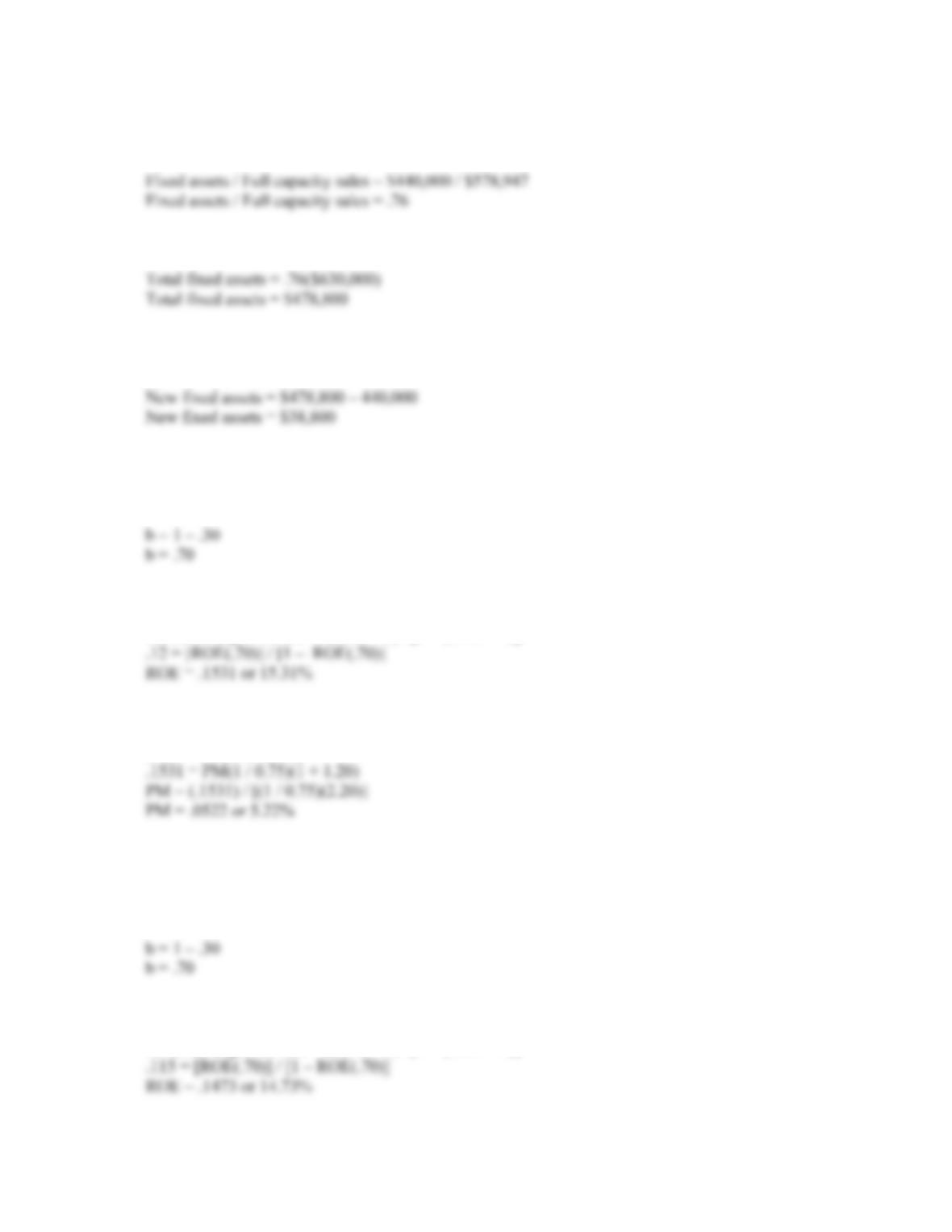B-38 SOLUTIONS
17. To find the new level of fixed assets, we need to find the current percentage of fixed assets to full
capacity sales. Doing so, we find:
Next, we calculate the total dollar amount of fixed assets needed at the new sales figure.
The new fixed assets necessary is the total fixed assets at the new sales figure minus the current level
of fixed assts.
18. We have all the variables to calculate ROE using the DuPont identity except the profit margin. If we
find ROE, we can solve the DuPont identity for profit margin. We can calculate ROE from the
sustainable growth rate equation. For this equation we need the retention ratio, so:
Using the sustainable growth rate equation and solving for ROE, we get:
Sustainable growth rate = (ROE × b) / [1 – (ROE × b)]
Now we can use the DuPont identity to find the profit margin as:
ROE = PM(TAT)(EM)
19. We have all the variables to calculate ROE using the DuPont identity except the equity multiplier.
Remember that the equity multiplier is one plus the debt-equity ratio. If we find ROE, we can solve
the DuPont identity for equity multiplier, then the debt-equity ratio. We can calculate ROE from the
sustainable growth rate equation. For this equation we need the retention ratio, so:
Using the sustainable growth rate equation and solving for ROE, we get:
Sustainable growth rate = (ROE × b) / [1 – (ROE × b)]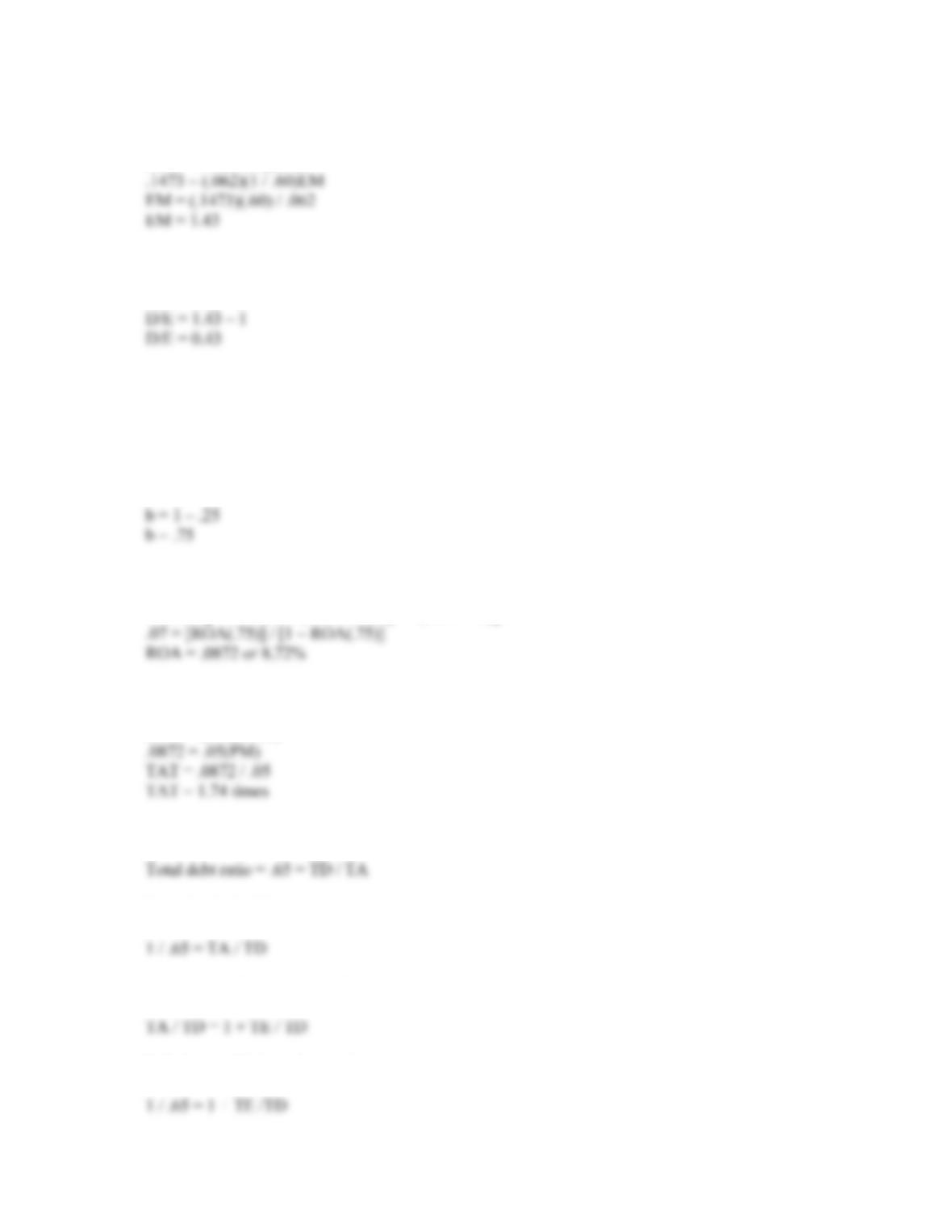CHAPTER 4 B-39
Now we can use the DuPont identity to find the equity multiplier as:
ROE = PM(TAT)(EM)
So, the D/E ratio is:
D/E = EM – 1
20. We are given the profit margin. Remember that:
ROA = PM(TAT)
We can calculate the ROA from the internal growth rate formula, and then use the ROA in this
equation to find the total asset turnover. The retention ratio is:
Using the internal growth rate equation to find the ROA, we get:
Internal growth rate = (ROA × b) / [1 – (ROA × b)]
Plugging ROA and PM into the equation we began with and solving for TAT, we get:
ROA = (PM)(TAT)
21. We should begin by calculating the D/E ratio. We calculate the D/E ratio as follows:
Inverting both sides we get:
Next, we need to recognize that
Substituting this into the previous equation, we get:

## Trusted by Thousands ofStudents

Here are what students say about us.